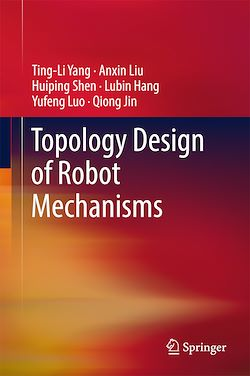# Topology Design of Robot Mechanisms

-English
236 Pages

Description

This book focuses on the topology theory of mechanisms developed by the authors and provides a systematic method for the topology design of robot mechanisms.

The main original theoretical contributions of this book include:

A. Three basic concepts

·         The “geometrical constraint type of axes” is introduced as the third element of the topological structure of a mechanism. When it is combined with the other two elements, the kinematic pair and the connection of links, the symbolic expression of the topological structure is independent of the motion positions (except for the singularity positions) and the fixed coordinate system (Chapter 2).

·         The position and orientation characteristic (POC) set is used to describe the POC of the relative motion between any two links. The POC set, derived from the unit vector set of the velocity of a link, is only depend on the topological structure of a mechanism. Therefore, it is also independent of the motion positions and the fixed coordinate system (Chapter 3).

·         The single open chain (SOC) unit is the base unit of the topological structure used to develop the four basic equations of the mechanism topology (Chapters 2, 4–6).

B. The mechanism composition principle based on the SOC units

This book proposes a mechanism composition principle, based on the SOC units, to establish a systematic theory for the unified modeling of the topology, kinematics, and dynamics of mechanisms based on the SOC units (Chapter 7).

C. Four basic equations

•        The POC equation of serial mechanisms with 10 symbolic operation rules (Chapter 4).

•        The POC equation of parallel mechanisms with 14 symbolic operation rules (Chapter 5).

•        The general DOF formula for spatial mechanisms (Chapter 6).

•        The coupling degree formula for the Assur kinematic chain (Chapter 7).

D. One systematic method for the topology design of robot mechanisms (Chapters 8–10)

Based on the three basic concepts and the four basic equations addressed above, this book puts forward a systematic method for the topology design of parallel mechanisms, which is fundamentally different from all existing methods. Its main characteristics are as follows:

•        The design process includes two stages: the first is structure synthesis, which derives many structure types; the second involves the performance analysis, classification and optimization of structure types derived from the first stage.

•        The design operation is independent of the motion positions and the fixed coordinate system. Therefore, the proposed method is essentially a geometrical method, which ensures the full-cycle DOF and the generality of geometric conditions of mechanism existence.

•        Each individual design step follows an explicit formula or the guidelines for design criteria, making the operation simple, feasible and reproducible. In addition, the topology design of the SCARA PMs is studied in detail to demonstrate the proposed method (Chapter 10).

Subjects

##### Artificial Intelligence

Informations